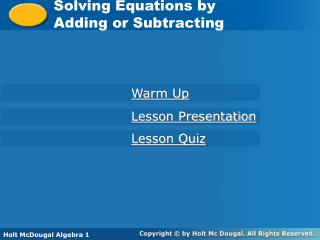DownloadDownload PresentationSolving Equations by Adding or Subtracting

# Solving Equations by Adding or Subtracting

Télécharger la présentation## Solving Equations by Adding or Subtracting

- - - - - - - - - - - - - - - - - - - - - - - - - - - E N D - - - - - - - - - - - - - - - - - - - - - - - - - - -
##### Presentation Transcript

1. Solving Equations by Adding or Subtracting Warm Up Lesson Presentation Lesson Quiz Holt McDougal Algebra 1

2. 3 1 2 2 2 3 3 3 3 2 3 Warm Up Evaluate. 1. + 4 2. 0.51 + (0.29) Give the opposite of each number. 3. 8 4.  Evaluate each expression for a = 3 and b = 2. 5. a + 5 6. 12  b 0.8 –8 14 8

3. Objective Solve one-step equations in one variable by using addition or subtraction.

4. Vocabulary equation solution of an equation

5. An equation is a mathematical statement that two expressions are equal. A solution of an equation is a value of the variable that makes the equation true. To find solutions, isolate the variable. A variable is isolated when it appears by itself on one side of an equation, and not at all on the other side.

6. Isolate a variable by using inverse operations which "undo" operations on the variable. An equation is like a balanced scale. To keep the balance, perform the same operation on both sides. Addition Subtraction Subtraction Addition

7. n – 3.2 = 5.6 Check Check It Out! Example 1a Solve the equation. Check your answer. n – 3.2 = 5.6 + 3.2+ 3.2 Since 3.2 is subtracted from n, add 3.2 to both sides to undo the subtraction. n = 8.8 To check your solution, substitute 8.8 for n in the original equation. 8.8 – 3.25.6 5.65.6 

8. –6 = k– 6 Check Check It Out! Example 1b Solve the equation. Check your answer. –6 = k –6 + 6+ 6 Since 6 is subtracted from k, add 6 to both sides to undo the subtraction. 0= k To check your solution, substitute 0 for k in the original equation. –6 0– 6 –6 –6 

9. 16 = m– 9 Check Check It Out! Example 1c Solve the equation. Check your answer. 16 = m –9 + 9+ 9 Since 9 is subtracted from m, add 9 to both sides to undo the subtraction. 25= m To check your solution, substitute 25 for m in the original equation. 16 25– 9 16 16 

10. 1 1 1 1 1 1 1 2 2 2 2 2 2 2 Since is added to d, subtract from both sides to undo the addition. – – d = 1 1 1 2 2 2 d + = 1 + 1 Check To check your solution, substitute for d in the original equation. Check It Out! Example 2a Solve the equation. Check your answer. d + = 1 1 1 

11. –5 = k+ 5 Check Check It Out! Example 2b Solve the equation. Check your answer. –5 = k +5 – 5– 5 Since 5 is added to k, subtract 5 from both sides to undo the subtraction. –10= k To check your solution, substitute –10 for k in the original equation. –5 –10+ 5 –5 –5 

12. 6 + t = 14 Check Check It Out! Example 2c Solve the equation. Check your answer. 6+t = 14 – 6– 6 Since 6 is added to t, subtract 6 from both sides to undo the addition. t = 8 To check your solution, substitute 8 for t in the original equation. 6 + 814 1414 

13. Remember that subtracting is the same as adding the opposite. When solving equations, you will sometimes find it easier to add an opposite to both sides instead of subtracting.

14. Check It Out! Example 4 A person's maximum heart rate in the highest rate, in beats per minute, that the person's heart should reach. One method to estimate maximum heart rate states that your age added to your maximum heart rate is 220. Using this method, write and solve an equation to find a person's age if the person's maximum heart rate is 185 beats per minute.

15. added to maximum heart rate 220 age is Check It Out! Example 4 Continued a+ r = 220 a + r = 220 Write an equation to represent the relationship. a + 185 = 220 Substitute 185 for r. Since 185 is added to a, subtract 185 from both sides to undo the addition. – 185– 185 a = 35 A person whose maximum heart rate is 185 beats per minute would be 35 years old.

16. Properties of Equality

17. Properties of Equality

18. Lesson Quiz Solve each equation. 1.r – 4 = –8 2. 3.m + 13 = 58 4. 0.75 = n + 0.6 5. –5 + c = 22 6. This year a high school had 578 sophomores enrolled. This is 89 less than the number enrolled last year. Write and solve an equation to find the number of sophomores enrolled last year. –4 45 0.15 27 s – 89 = 578; s = 667